# Top 10 Excel functions you need to know in 2023These are the top 10 excel functions that you need to learn in 2023. With these 10 functions you can unlock the power of Excel. Functions are the driving force behind Excel. They allow you to turn info into insights. Functions in Excel allow you to interpret and alter your data according to the conditions you set.

However, if you’re an Excel pro, this list isn’t for you. Our list goes over the basics — stuff everyone needs to know — and a few new things recently added by Microsoft.

Follow along and try these out yourself. The example data set is available here! We’ll be assuming the role of a car dealership owner.

### The 10 Excel functions to use in 2023

#### 1. IF

First of all, let’s start with a simple yet very useful function. The IF function allows you to compare two values and receive an output based on the answer.

##### Syntax
=IF(logical argument,value if true,value if false)

In our example, I want to know if the price we’ve listed for each car is greater than the cost we paid for it.

So our function is =IF(E2>F2,”OK”,”Raise Price”).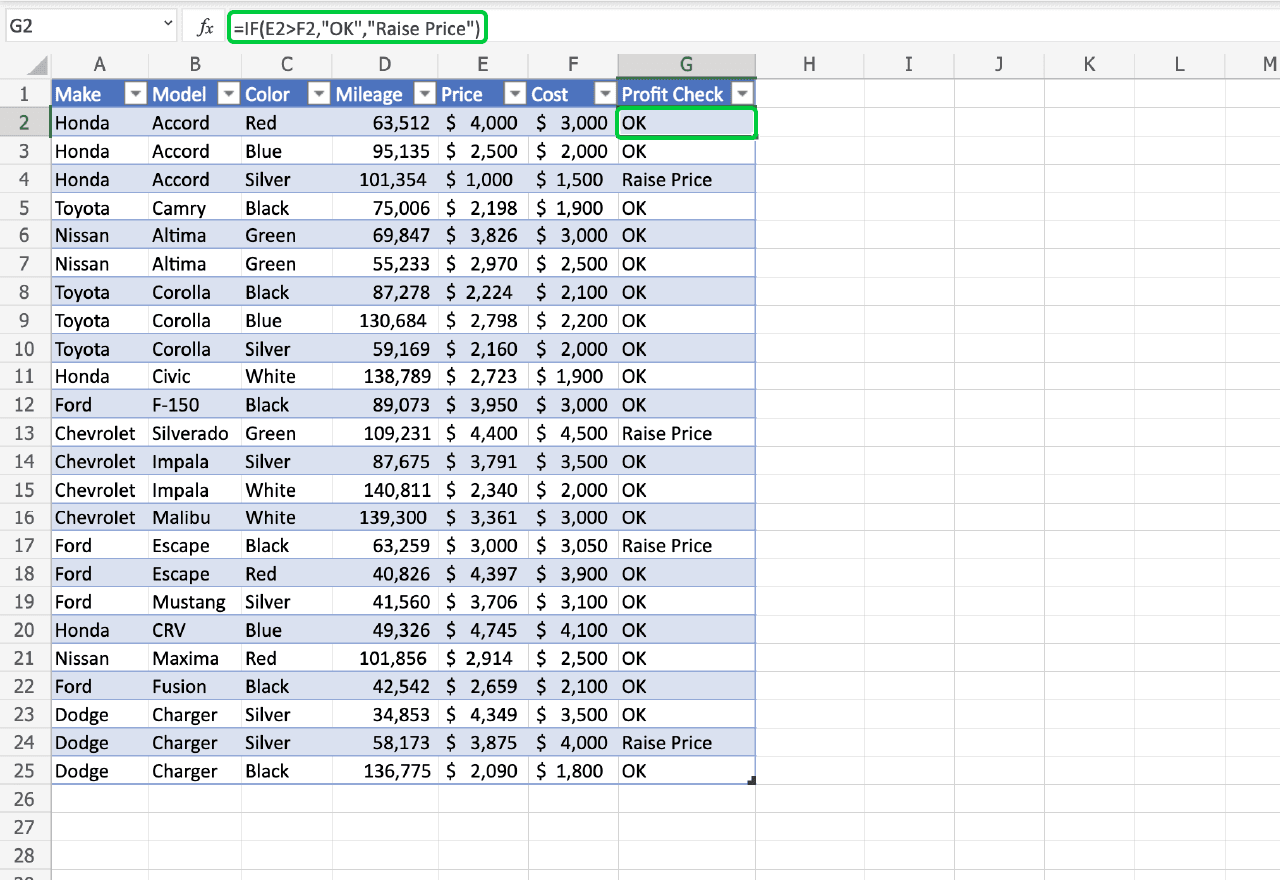Basically, the =IF function allows you to set values for true and false. Here we’ve set it to read “OK” if our price is higher than our cost and “Raise Price” if we’ll lose money at the current price.

Let’s label our new column Profit Check. Include the quote-marks if you want to use a string of letters. Because if you don’t, Excel will think you’re entering a function and it won’t recognize it, creating a #NAME? error for unrecognized text.

#### 2. SUM

Next up is the SUM function. It does exactly what you’d expect it to do. It sums the items in a specified range.

It’s usually the first function you learn when you open up Excel. It’s a great stepping stone for understanding how functions work.

##### Syntax
=SUM(number1,number2,…)

Remember that mathematics symbols can be inserted to change the function performed from adding to your desired operation.

In our example, I want to know what our total profit will be if we sell all of our current inventory. This means that my function will be the range of our list price minus the range of our cost, written as =SUM(E2:E25-F2:F25).

As a result, the SUM function automatically totals the two ranges and subtracts the total cost from the total list price.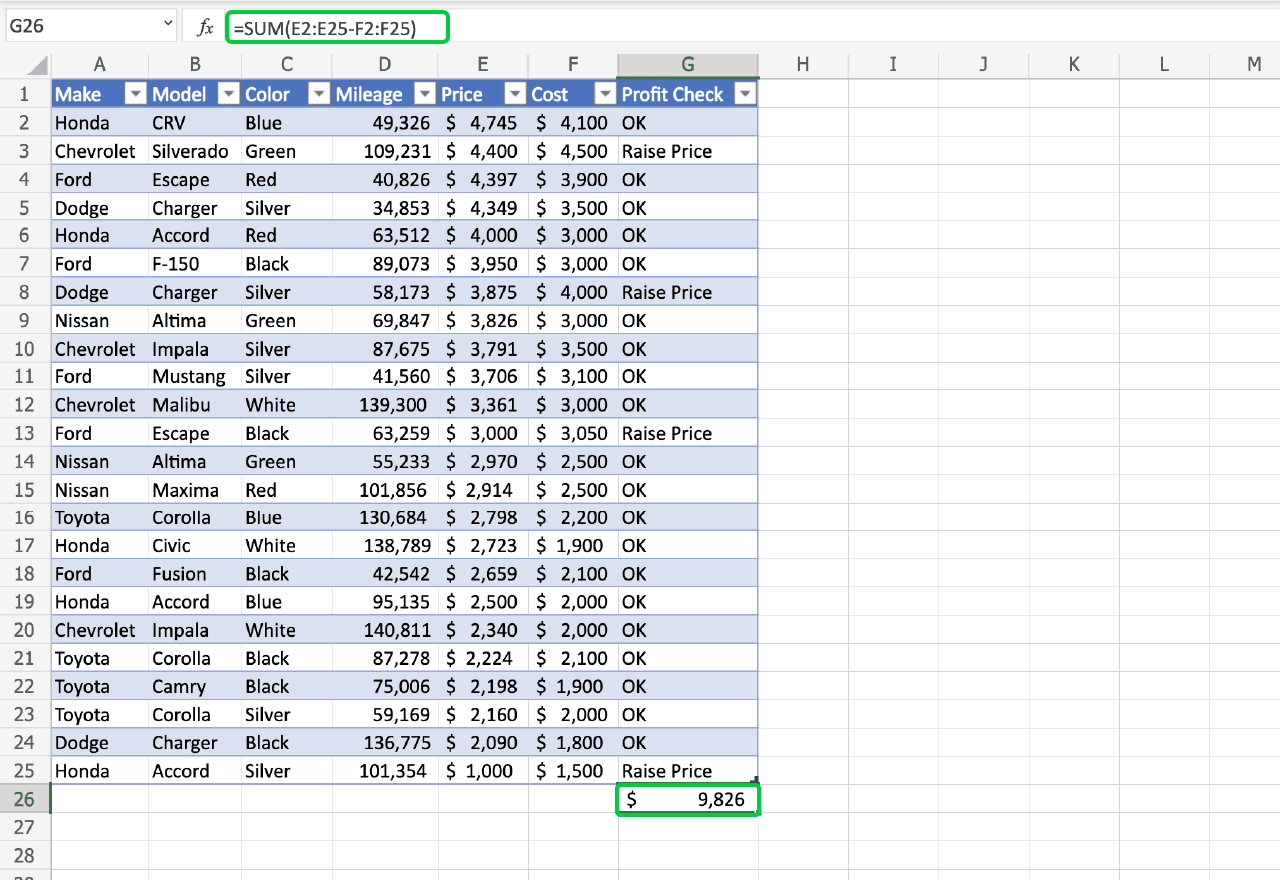While SUM is simple — it’s just a basic math equation — It is a great time saver and shouldn’t be overlooked. It’s a quick way to understand your dataset.

#### 3. SUBTOTAL

Similar to SUM, SUBTOTAL performs a function on a group of numbers.

Its power, however, comes from its modifiers. Depending on the modifier you include, SUBTOTAL can display the average, count, or rage of your data set, among other things.

The list of modifiers is available on Microsoft’s website.

##### Syntax
=SUBTOTAL(function modifier,number1,number2,…)

We want to know the average cost and list price for our inventory.

So our functions are =SUBTOTAL(101,E2:E25) and =SUBTOTAL(101,F2:F25).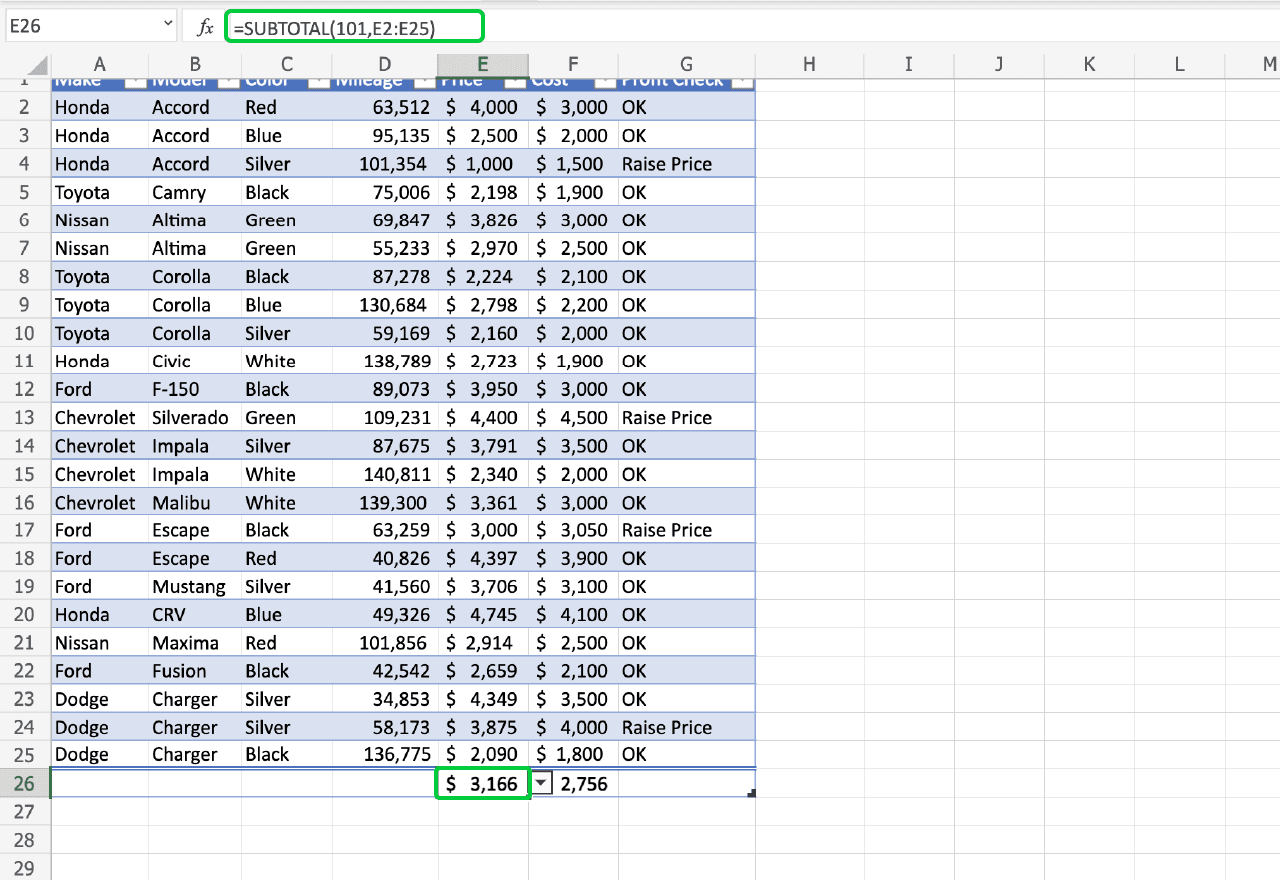When the SUBTOTAL function is used at the bottom of a table, a dropdown menu appears that lets you cycle through each of the operations it performs, making analysis a snap.

#### 4. CONCAT

The CONCAT function joins, or concatenates, the inputs. It’s that simple.

You might have a list of first names and a list of last names, the CONCAT function will create a list of full names for you.

##### Syntax
=CONCAT(input1,input2,…)

If you want a space between elements, include a space between quote marks in your input list. It will look like this: (input1,” “,Input2).

We want to make a column with the title of each car to post on our website. Because color, make, and model are all separate cells, we’re going to use the CONCAT function to make one cell with all of that information.

To do that we’ll write =CONCAT(C2,” “,A2,” “,B2). Make sure to include spaces in quotes between each element.

Let’s call our new column Sticker Name. Now we have a list of titles that we can hand over to our webmaster.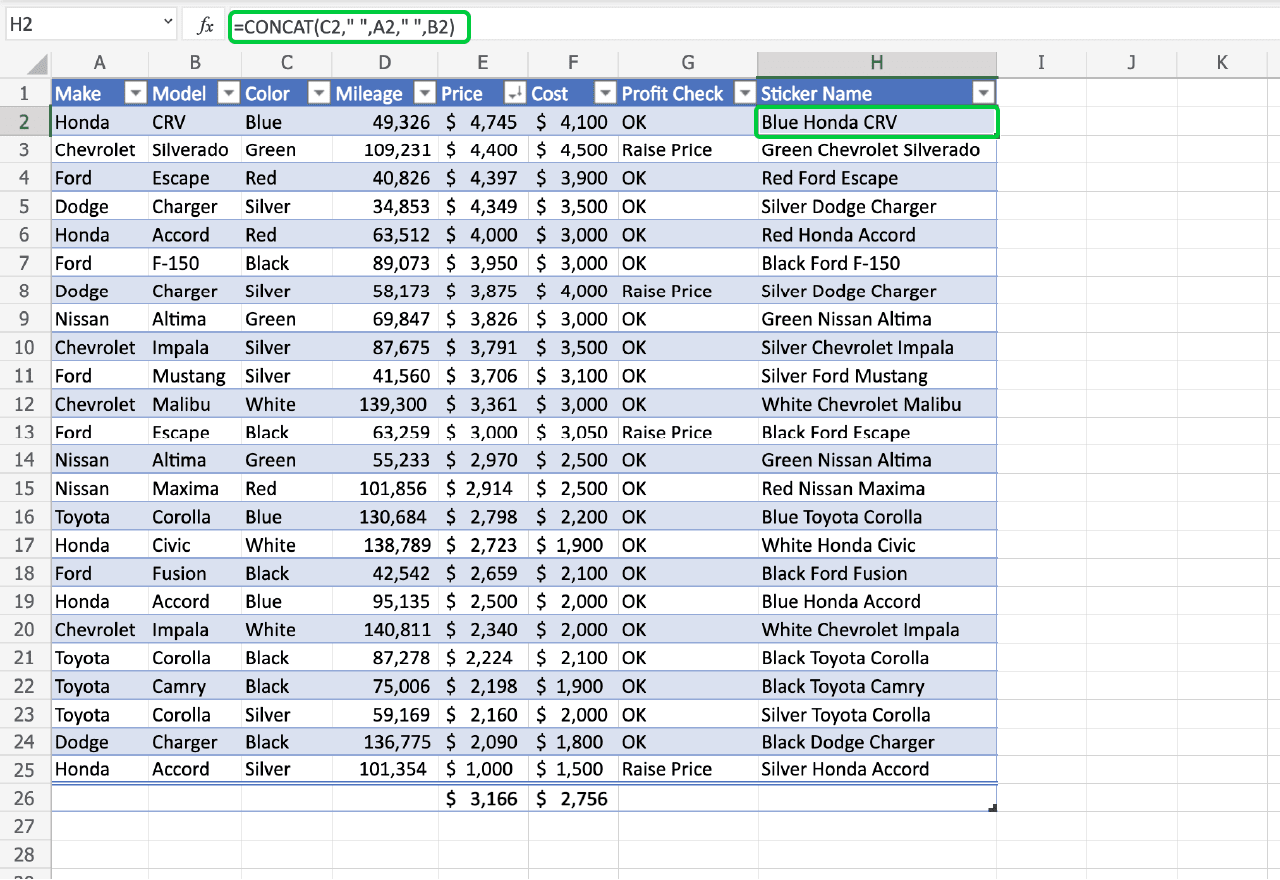#### 5. COUNTA

Similar to some previous Excel functions listed, the COUNTA function is another basic, but useful function. It simply counts. COUNTA will total the number of nonempty cells in a range.

It doesn’t matter if the cell contains a number, a string of letters, or an error, COUNTA will count it. On the other hand, if you only want a count of cells with number values, you can use =COUNT.

##### Syntax
=COUNTA(first cell in range:last cell in range)

If we want to have a running total of our inventory, we can use the COUNTA function for an entire Column.

To do that, we’ll write =COUNTA(A:A)-1 off to the side of our table. We include the minus one because the COUNTA function is counting our column label which we want to exclude.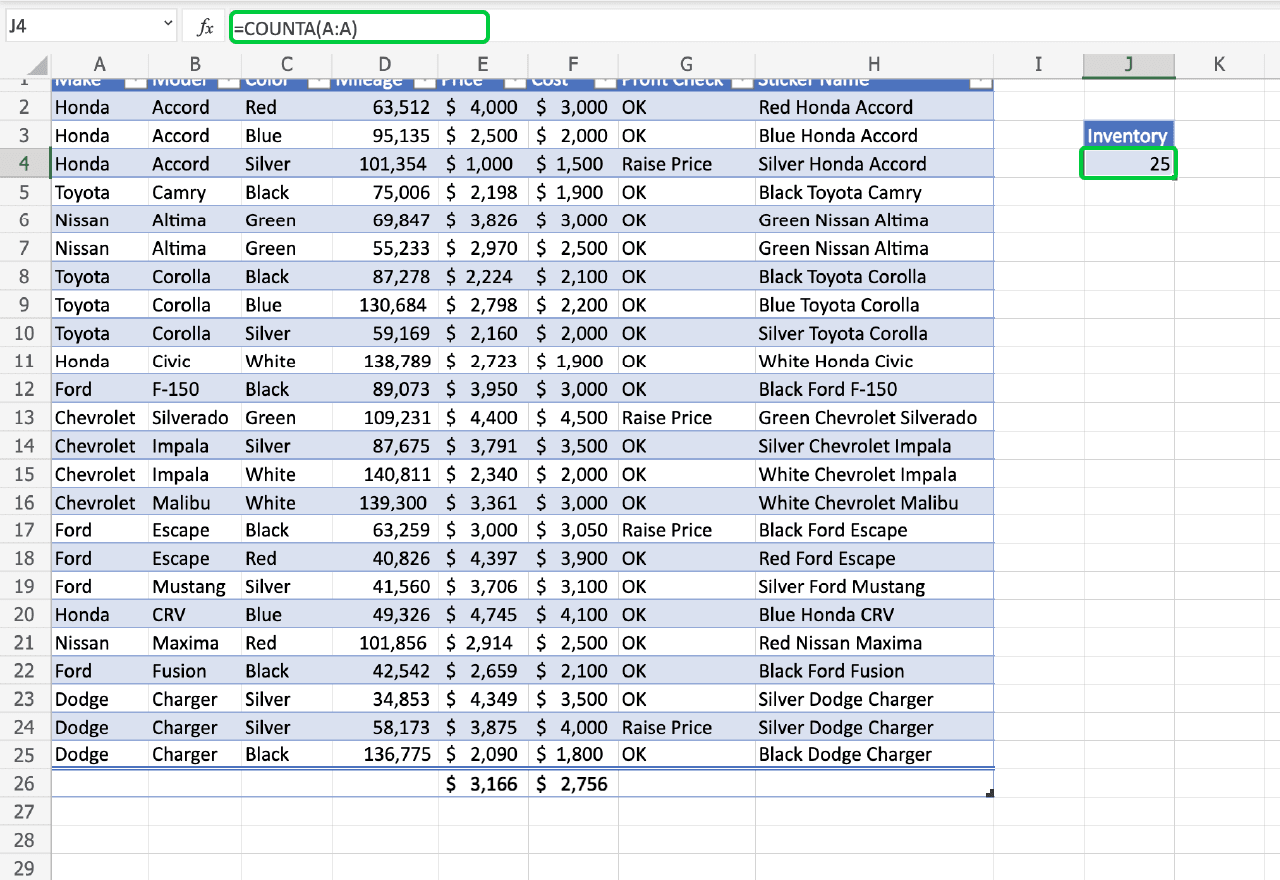Because COUNTA only counts cells with something in them, we can count the whole column and only get back the number of rows with information in them.

Now, when we update our inventory list, our inventory counter will update automatically. Put a label in front of our formula so you remember what the number represents.

#### 6. COUNTIF

COUNTIF is related to COUNTA.

COUNTIF is a conditional counter, which means it only counts cells that fulfill an argument you give it.

##### Syntax
=COUNTIF(first cell in range:last cell in range,logical argument)

In this example, what if we wanted to know how many of the various brands of cars we have in stock. To do that we can use the COUNTIF function. Column A as our range. For the argument, if we put the model name in quotes, COUNTIF will only count the cells whose content matches the brand we’re interested in.

For example, our Honda counter is written =COUNTIF(A:A,”Honda”). We can create one for each of the models we carry, and make sure to label them.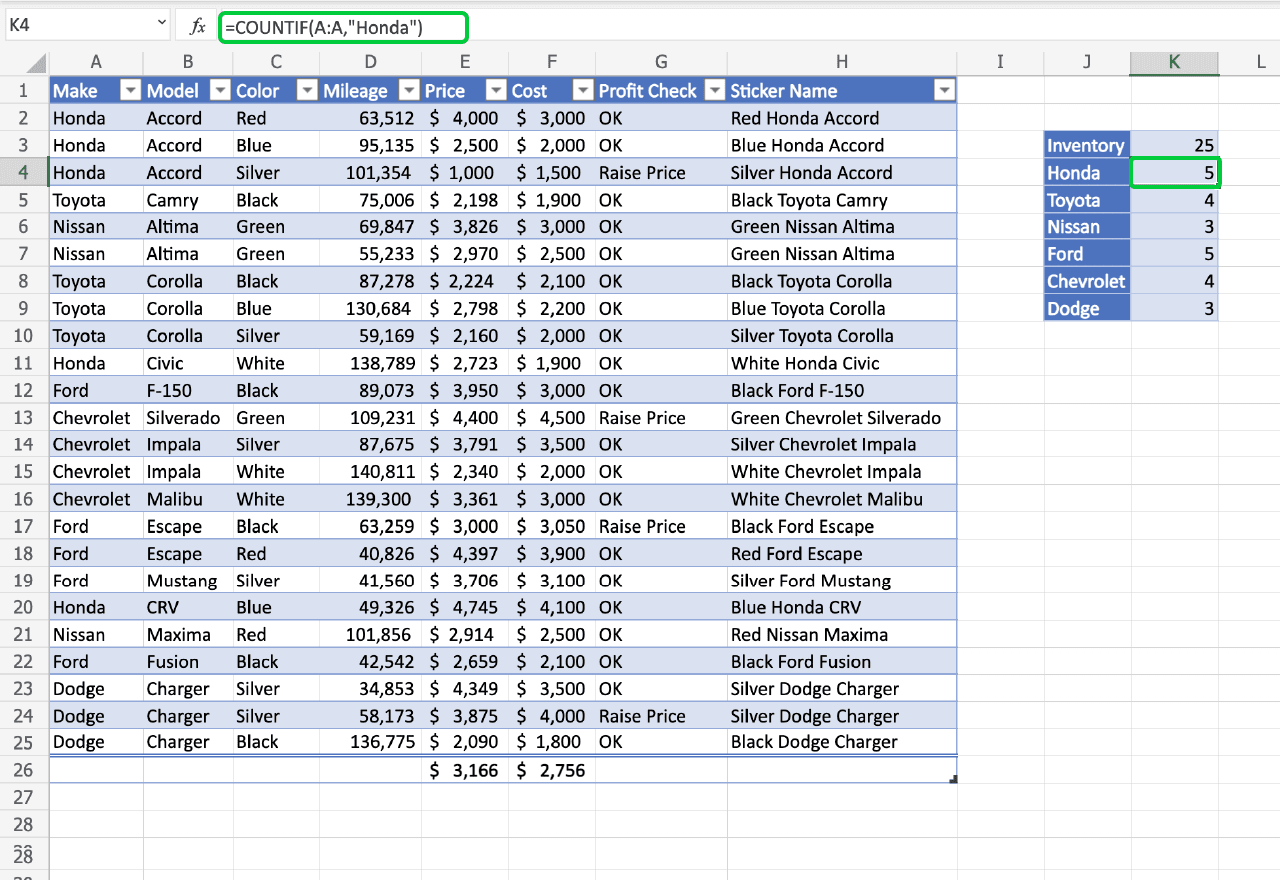Now we have a granular view of our inventory to go along with our general count.

#### 7. UNIQUE

What if we don’t know all of the types of models we have in stock?

The UNIQUE function is useful in that situation. It creates a list of all of the unique values in a data set. It can also be used to make a list of values that only appear once.

##### Syntax
=UNIQUE(Array,By Column T/F, Exactly Once T/F)

By default it assumes that our data is organized in rows, and we want a list of the unique values in an array, but you can change that by setting the respective modifiers to true.

For our car dealership example, the UNIQUE function can replace the manually typed make names. In the first cell we type =UNIQUE(A2:A25). You don’t have to include the modifiers, they’ll automatically be set to false.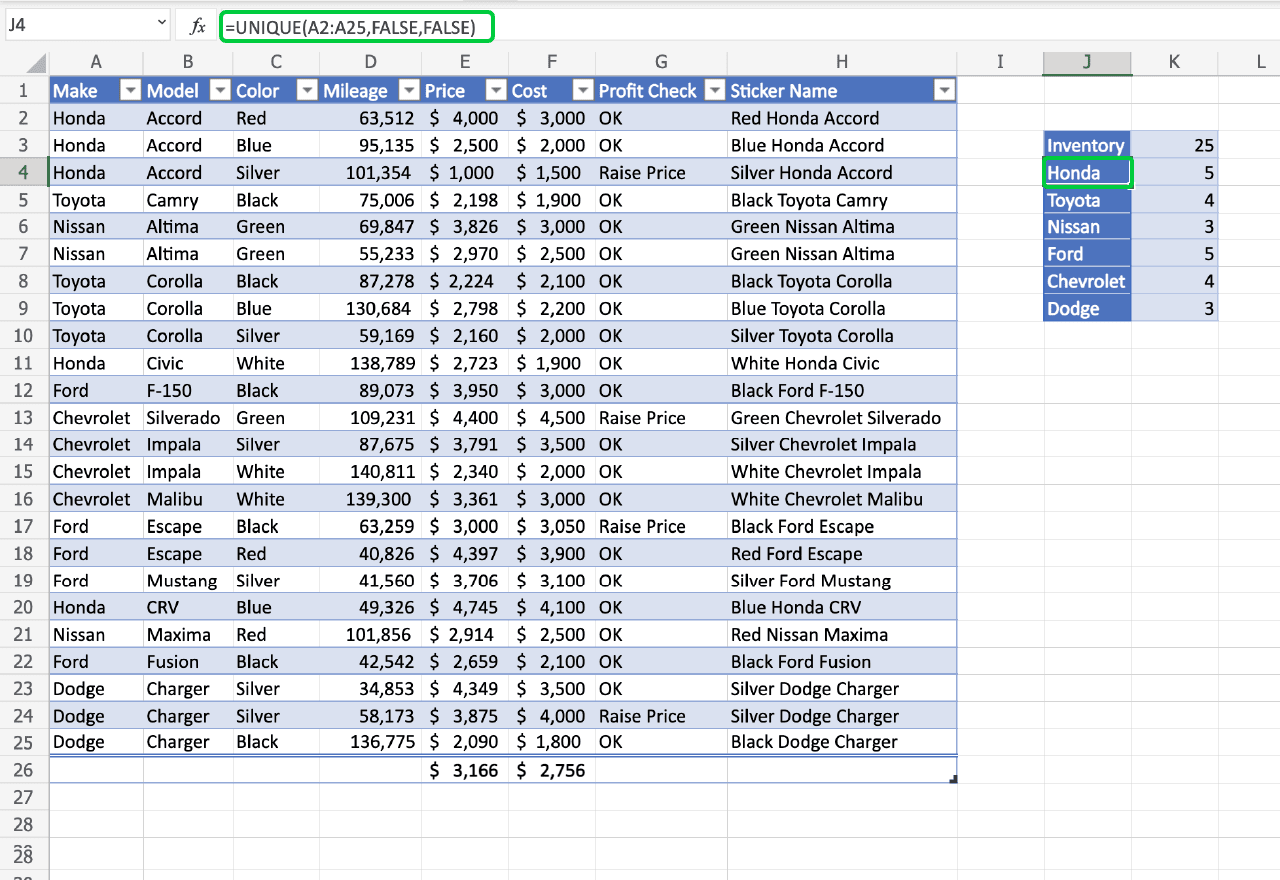Now we’ve confirmed which brands we have in our inventory. Each brand is displayed once in our function’s list.

At this point we can update our COUNTIF functions by changing the brand name with the cell containing the brand in our new list. Now, anytime we reorder our list, or change a brand name, our inventory counter will update automatically.

#### 8. SEQUENCE

On its surface, the SEQUENCE function doesn’t seem like it would be very useful. It only creates a list of numbers.

But when combined with other functions, it becomes a powerful tool.

##### Syntax
=SEQUENCE(number of row, number of columns,starting number, step size)

The SEQUENCE function assumes, if left blank, that columns, start number, and steps should be set to one.

Let’s assume we need to give each car in our list a unique identifying number. SEQUENCE can help us out. How can we make our sequence dynamic so that it automatically updates when we add or remove a vehicle from our stock? What if we reference our dynamic inventory counter from earlier to populate our sequence.

That would look like this: =SEQUENCE(L3). Let’s insert a new column on the left and title it ID Number.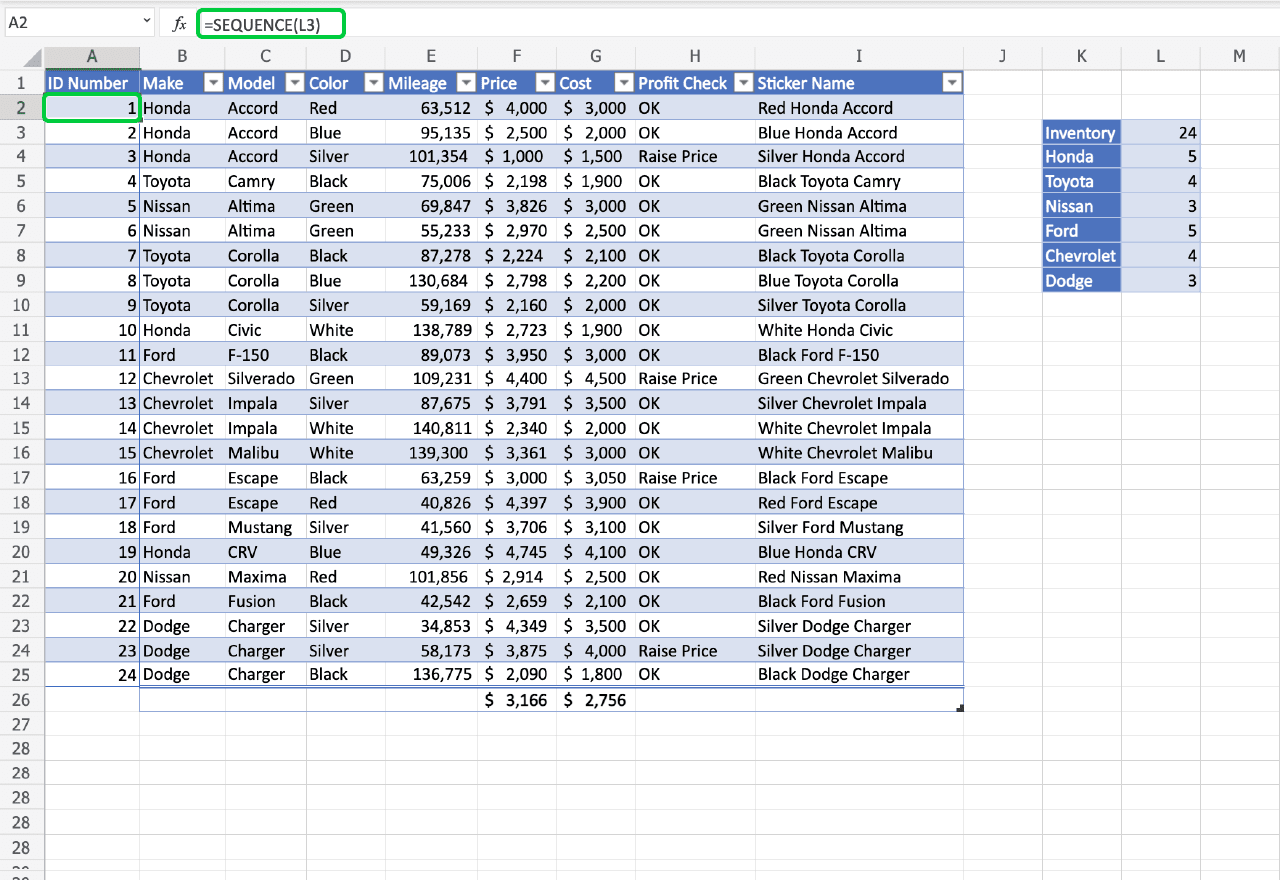Now each of our vehicles has a unique ID Number, that sets us up nicely for our next function.

#### 9. XLOOKUP

If you’re familiar with Excel you might know the function VLOOKUP. Well, XLOOKUP is a new and improved version of VLOOKUP. If you want to read about VLOOKUP, check out our previous blog post here.

XLOOKUP works by matching a source to a cell in an array and returning information from a parallel array.

##### Syntax
=XLOOKUP(Lookup value,lookup array, return array,error message)

Back at the car dealership, let’s use XLOOKUP to find the price of a vehicle based on its ID Number. To do that we’ll write the ID Number we want to find in the cell above our XLOOKUP function.

That way we can reference the cell in our formula and easily change the ID Number we’re looking for. Our formula will be =XLOOKUP(L11,A:A,F:F).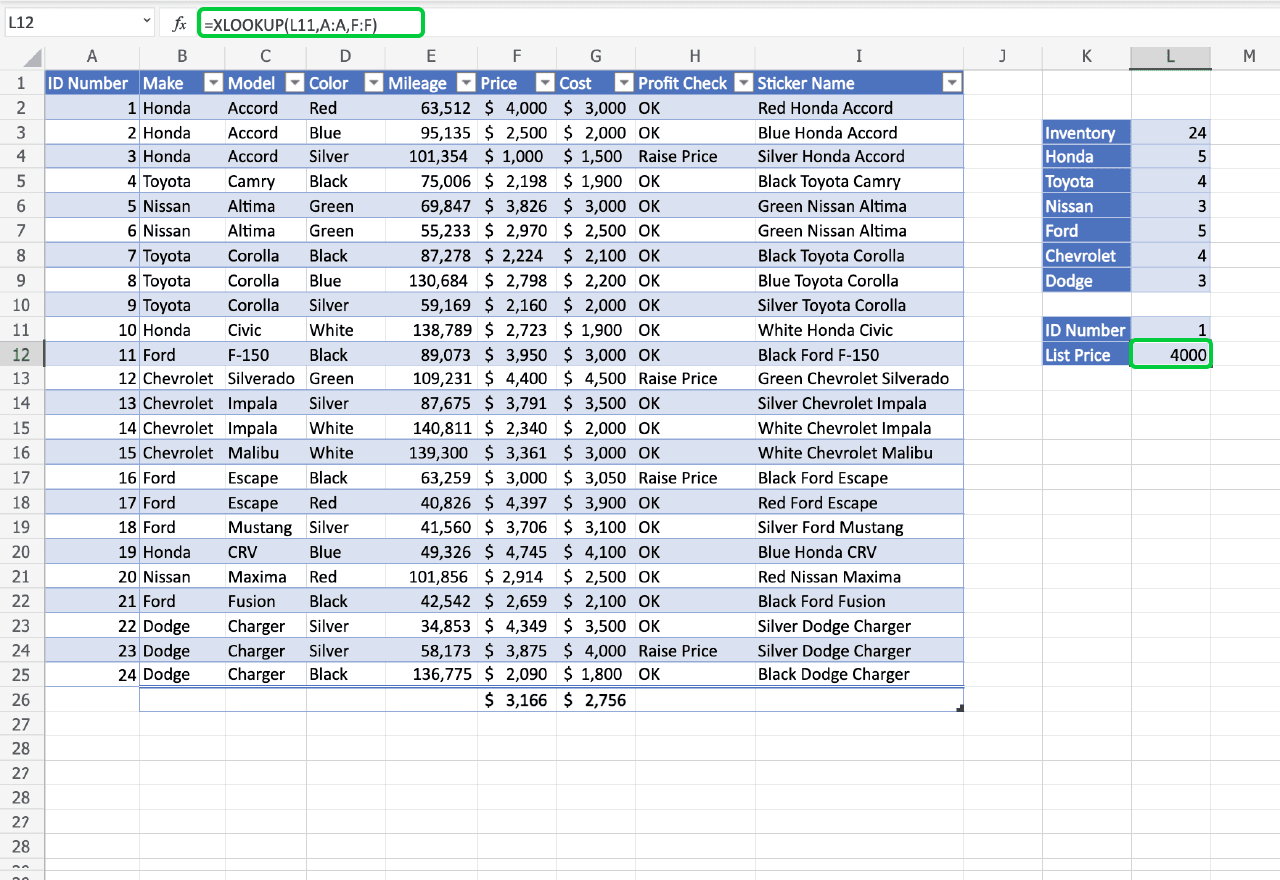If you go in and change the ID Number, the List Price will update automatically. This is a great tool for large datasets. Especially if everything has an ID or serial number.

#### 10. FILTER

Now lastly, my favorite function, FILTER.

The FILTER function is like combining XLOOKUP and IF functions. It displays results based on conditions you provide.

##### Syntax
=FILTER(Array,Include If,error message)

Searching our inventory for certain colors becomes easy with the FILTER function. We’ll write the color we’re interested in first so we can reference it in our function.

In the cell below that we’ll write our function, =FILTER(I2:I25,D2:D25=L14). We’re just pulling up the Sticker name in this example, but you can have it display as large of an array as you’d like.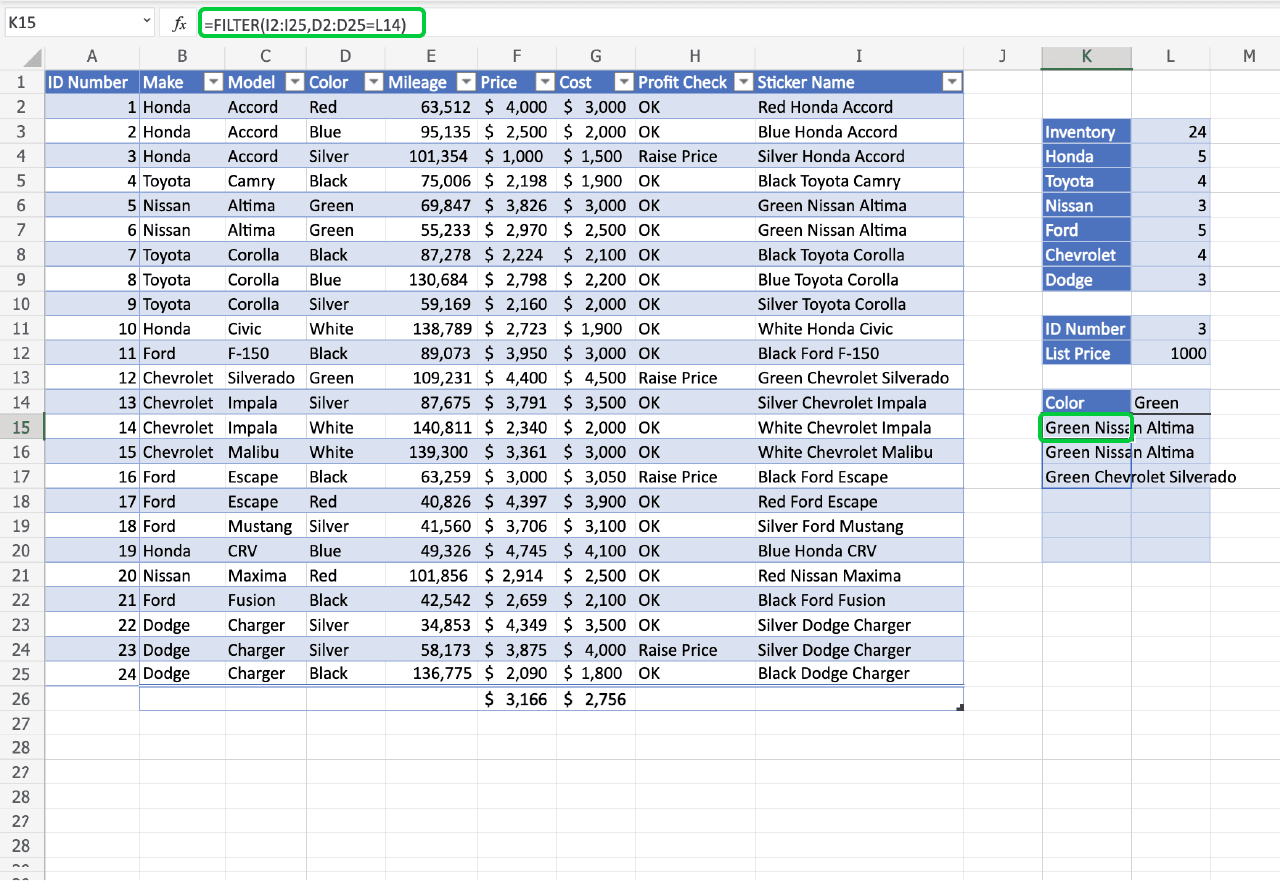Now you can type any color and pull up available models in that color. A great tool for salespeople who wanted to tell a client what is available in the color they want.

### Final thoughts

With just these 10 functions we’ve created a handy little spreadsheet, saving our small business time. This isn’t a comprehensive list. Many more powerful Excel formulas exist.

To read up on some of them, check out the rest of our blog.